# Introduction To Trigonometry - Olympiad Level MCQ, Class 10 Mathematics

## 25 Questions MCQ Test Olympiad Preparation for Class 10 | Introduction To Trigonometry - Olympiad Level MCQ, Class 10 Mathematics

Description
Attempt Introduction To Trigonometry - Olympiad Level MCQ, Class 10 Mathematics | 25 questions in 25 minutes | Mock test for Class 10 preparation | Free important questions MCQ to study Olympiad Preparation for Class 10 for Class 10 Exam | Download free PDF with solutions
QUESTION: 1

Solution:
QUESTION: 2

Solution:
QUESTION: 3

###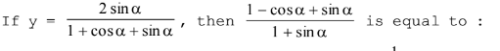Solution:
QUESTION: 4

cot 36° cot 72° is equal to :

Solution:
QUESTION: 5

The value of cos2 15° – cos2 30° + cos2 45° – cos2 60° + cos2 75° is :

Solution:
QUESTION: 6

If x = sin2 θ cos θ and y = cos2 θ sin θ, then :

Solution:
QUESTION: 7

If x = secθ – tanθ and y = cosecθ + cotθ, then xy + 1 is equal to :

Solution:
QUESTION: 8

If 5 sinθ = 3, then secθ tanθ /secθ – tanθ is equal to :

Solution:
QUESTION: 9

The value of the expression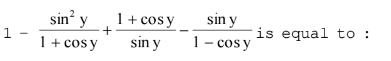Solution:
QUESTION: 10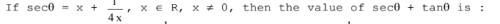Solution:
QUESTION: 11

Given that sin A=1/2 and cos B=1/√2 then the value of (A + B) is:

Solution:
QUESTION: 12

If m = tanθ + sinθ and n = tanθ – sinθ, then (m2 – n2)2 is equal to :

Solution:
QUESTION: 13

If x = a cos θ + b sin θ and y = a sin θ – b cos θ then a2 + b2 is equal to :

Solution:
QUESTION: 14

If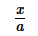cosθ +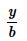sinθ + 1 = 0 andsinθ –cosθ – 1 = 0 then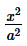+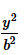is equal to :

Solution:
QUESTION: 15

ABC is a triangle, right angled at A. If the length of hypotenuse is 2 √2 times the length of perpendicular from A on the hypotenuse, the other angles of the triangle are :

Solution:
QUESTION: 16

If sin A + cos A = m and sin3A + cos3A = n, then

Solution:
QUESTION: 17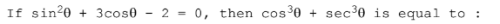Solution:
QUESTION: 18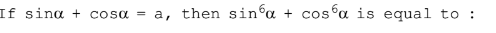Solution:
QUESTION: 19

The quadratic equation whose roots are sin 18° and cos 36° is :

Solution:
QUESTION: 20

If cosθ + sectθ = 2, then the value of cos2θ + sec2θ is :

Solution:
QUESTION: 21

If sin (A – B) = cos (A + B) =1/2, then the values of A and B lying between 0° and 90° are respectively:

Solution:
QUESTION: 22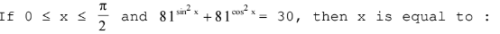Solution:
QUESTION: 23

If m2 + m'2 + 2mm' cosθ = 1, n2 + n'2 + 2nn' cos θ = 1, and mn + m'n' + (mn' + m'n) cos θ = 0, then m2 + n2 is equal to :

Solution:
QUESTION: 24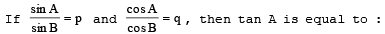Solution:
QUESTION: 25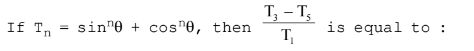Solution:Use Code STAYHOME200 and get INR 200 additional OFF Use Coupon Code